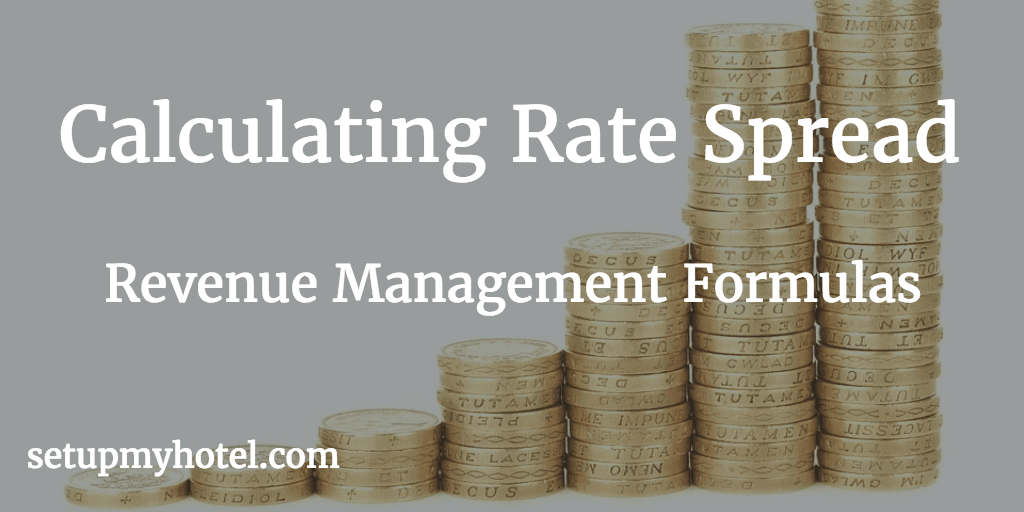# Calculating The Room Rate Spread

Rate Spread is another important matrix used by revenue management team in large hotels or by the front office manager in smaller hotel operations.

The value for rate spread is derived from various room types in the hotel in order to make essential yield decisions by the hotel management. Rate Spread is calculated by taking the difference between Potential Average Double Rate and Potential Average Single Rate

The Formula for Calculating Rate Spread

Rate Spread = Potential Average Double Rate - Potential Average Single Rate

Potential Avg. Double Rate:

Potential Avg. Single Rate:

Example 1:

Potential Average Single Rate   = 150.00

Potential Average Double Rate  = 225.00

Rate Spread of the hotel      = 225.00 - 150.00

= 75.00

Example 2:

Potential Average Double Rate  = 109.00

Potential Average Single Rate   = 99.00

Rate Spread of the hotel      = 109.00 - 99.00

= 10.00

Potential Average Double Rate For the Month = 158.88

Potential Average Single Rate For the Month  = 129.69

Rate Spread of the hotel      = 158.88 - 129.69

= 29.19